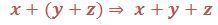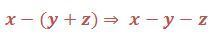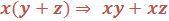• No products in the cart.

FREE
0

# BODMAS Rule

Example- Solve (12+14)of16 Solution-  (12+14)of16 Step 1: Solving the fraction inside the bracket first- 12+14=34   Step 2: Now the expression will be 34of16 34×16 =12 …## Course Description

Example- Solve (12+14)of16 Solution-  (12+14)of16 Step 1: Solving the fraction inside the bracket first- 12+14=34   Step 2: Now the expression will be 34of16 34×16 =12

### SIMPLIFICATION OF BRACKETS

Simplification of brackets in an expression means expansion of brackets. We can remove brackets from an expression by expanding them by multiplication. We use distributive law of multiplication over addition or subtraction. Generally, it can be written as:Notes: The order of brackets to be simplified is (), {}, []. Example 2: Expand and simplifySolution:
1. The terms inside the bracket are like terms. We can solve this by two methods.
Method 1:  Add the terms inside the bracket and multiply the number outside bracket with the sum.Method 2: Multiply the number outside the bracket with each term inside the bracket and add the products.1. Here the terms are unlike. This can be simplified by multiplying the number outside the bracket with the terms inside the bracket and add the products.Step 1: Simplify the terms inside {}. Step 2: Simplify {} and operate with terms outside the bracket. 1800÷10(126)+(2412) 1800÷10{6+12} 180{18} =3240(ii) 12[{2(1+2)}10]< Step 1: Simplify the terms inside () followed by {}, then []. Step 2: Operate terms with the terms outside the bracket.Condition RuleOpen the bracket and add the terms.Open the bracket and multiply the negative sign with each term inside the bracket.(All positive terms will be negative and vice-versa)Multiply term outside with each term inside the bracket